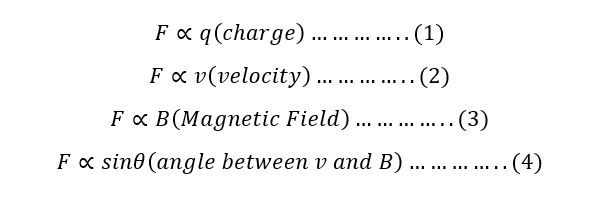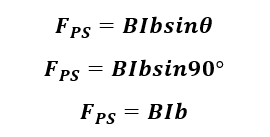# Magnetic FieldMagnetic Field

So, you might be thinking what is magnetic field? So the answer is like this; You might have a magnet and then place it around the iron fillings on paper and simply tap the paper. You will see that iron fillings get arranged in a certain pattern and this pattern is due to the field know as magnetic Field. To be more clear here is the image:The concept that the current carrying conductor produces a magnetic field in the periphery of itself was first found by the Danish Scientist. He did a simple experiment to show that magnetic field are indeed produced due to moving charge.

What he did was, he just placed a compass needle below the current carrying wire and to his surprise he found out that the magnetic needle deflected. This way he demonstrated that magnetic fields are the origin of moving charges.

Force Experienced by a moving charge in a Magnetic field

Let us consider a particle of charge q moving with a velocity v in the region of magnetic field B at an angle of theta with the magnetic field. Then from the Lorentz interaction, this moving charge will experience a force. Experimentally it has been found that;So combining all we get;Since k=1, we haveThis is the force with which a moving charge experiences when placed in a magnetic field.

In vector form it is the cross product of velocity and Magnetic Field.SPECIAL CASES:Hence, moving charge won’t experience any force.Hence, moving charge will experience maximum force.

DIRECTION OF FORCE

The direction of force is basically perpendicular to the plane of v and B as mentioned from the vector form of force. In general, the direction of the force experienced by a moving charge in a magnetic field is given by Fleming’s Left Hand Rule. The rule states that;

If your curl your first three fingers of your left hand perpendicular to each other such that index finger points in the direction of magnetic field, middle finger in the direction of conventional current then the thumb represents the direction of force. i.e.Force Experienced by a current carrying conductor in a magnetic field

Let us imagine a conductor of length l having cross sectional area A which is carrying current I is placed in a magnetic field B at and angle theta. Let n be the no of electrons per unit volume, then,In vector form,Torque Experienced by current carrying rectangular loop in Uniform Magnetic field

Let us consider a loop of length l and breadth b carrying current I. The normal of the loop makes an angle of theta with the magnetic field.

This is based on the principle that when a current carrying loop is placed in a uniform magnetic field then it experiences a force and this force causes it to rotate producing torque.Here the force experienced by the arm PS(l) is given byThen force experienced by arm QR(l) is given bySince the direction of these forces are opposite and have same magnitude these two forces, they cancel each other. The direction of them is given in the figure 3.24.

Then The force experienced by the arm RS is given by,Similarly, the force experience by arm PQ is also same as that of PS but is opposite in direction.

Then these forces tend to rotate the loop along the vertical axis.

Then, the net torque experienced by the loop is given by,If the coil has N number of turns then,This the torque experienced by the loop in a uniform magnetic field.

If theta is the angle between the plane of the magnetic field and the plane of the coil then,SPECIAL CASES: